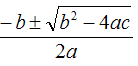# Visual Basic 2010 GuideIf...Then...Else

## Simple If

This is our first example of a control statement. The If statement allows you to specify conditions under which some lines of your code will be run. Copy and run the following program,

```Dim score As Integer Console.Write("Enter the score: ") score = Console.ReadLine() If score > 60 Then score += 10 Console.WriteLine("Score = {0}", score) Console.ReadLine()```

Study the line with the If statement carefully. When there is only one line of code to run if the statement is true, it can be written in a single line as shown above.

## Simple Block If

It is more likely that you will want to execute several lines of code with your if statements. In such cases, you should write your statement as shown in the next sample. Overtime is paid at double time for hours worked over 40.

```Dim overtime As Integer = 0 Dim hours As Integer Dim pay As Double Console.Write("Enter the number of hours worked: ") hours = Console.ReadLine() pay = hours * 3.75 If hours > 40 Then    overtime = hours - 40    pay += overtime * 3.75 End If Console.WriteLine("Total pay is £{0}", pay) Console.ReadLine()```

Notice now that we have the End If statement to mark the end of our block of code.

## If...Then...Else

We will sometimes want to be able to execute one line of code if a condition is true and something else if it is not. In the following example we determine whether someone has passed or failed an examination.

```Dim score As Integer Console.Write("Enter the score: ") score = Console.ReadLine() If score > 40 Then    Console.WriteLine("You have passed the exam") Else    Console.WriteLine("You have failed the exam") End If Console.ReadLine()```

The keyword, Else is used to introduce the code to run when the condition is not met.

## If..Then...Else If...Then...Else

We can also use the If statement to check a series of conditions. The following program is used to calculate a grade from an exam score.

```Dim grade As String Dim score As Integer Console.Write("Enter the score: ") score = Console.ReadLine() If score >= 75 Then    grade = "A" ElseIf score >= 65 Then    grade = "B" ElseIf score >= 55 Then    grade = "C" Else    grade = "U" End If Console.WriteLine("Your grade for the examination is {0} ", grade) Console.ReadLine()```

Consider here that, if you enter an A grade score, it will be greater than 65 and 55 as well as being greater or equal to 75. The first condition that is met will be executed. Notice also that we can use the else statement to add a clause to cover the situation when none of the other conditions are met.

## Having A Go

1. Write a program that reads an employee's number, their hourly rate and the number of hours worked in that week. Hours over 40 are paid at time and a half. Calculate and display the wages due to this person.
2. Write a program that reads two numbers and examines the relationship between them. Output one of the following messages,

A is greater than B
B is greater than A
A and B are equal
3. Write a program to find the two roots of the quadratic equation ax2 + bx + c = 0 using the formula,The user inputs values for a, b and c. If b2 - 4ac is negative, then the equation has no roots. If b2 - 4ac=0, the equation has only one root.